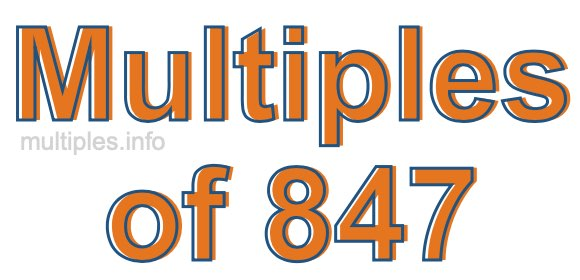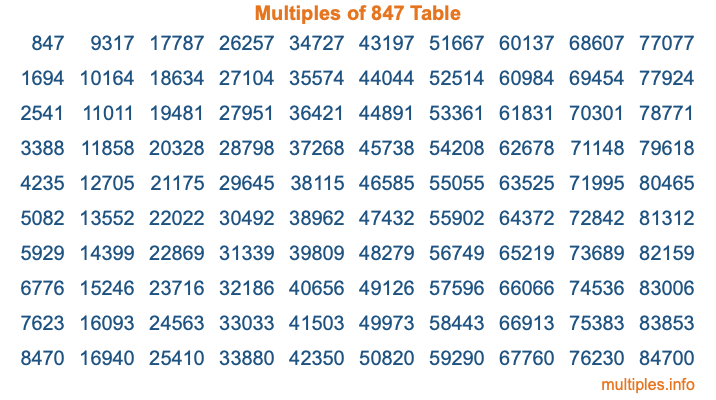Multiples of 847Welcome to the Multiples of 847 page. Here we will first teach you everything you will ever need to know about the multiples of 847, and then give you a study guide summary of everything we taught you to make sure you remember it all. Use this page to look up facts and learn information about the multiples of 847. This page will make you a multiples of eight hundred forty-seven expert!

Definition of Multiples of 847
Multiples of 847 are all the numbers that when divided by 847 equal an integer. Each of the multiples of 847 are called a multiple. A multiple of 847 is created by multiplying 847 by an integer.

Therefore, to create a list of multiples of 847, you start with 1 multiplied by 847, then 2 multiplied by 847, then 3 multiplied by 847, and so on for as long as you want. Thus, the list of the first five multiples of 847 is 847, 1694, 2541, 3388, and 4235. To see a larger list of multiples of 847, see the printable image of Multiples of 847 further down on this page. We also have a category where you can choose any nth multiple of 847.

Multiples of 847 Checker
The Multiples of 847 Checker below checks to see if any number of your choice is a multiple of 847. In other words, it checks to see if there is any number (integer) that when multiplied by 847 will equal your number. To do that, we divide your number by 847. If the the quotient is an integer, then your number is a multiple of 847.

Is  a multiple of 847?

Least Common Multiple of 847 and ...
A Least Common Multiple (LCM) is the lowest multiple that two or more numbers have in common. This is also called the smallest common multiple or lowest common multiple and is useful to know when you are adding our subtracting fractions. Enter one or more numbers below (847 is already entered) to find the LCM.

Check out our LCM Calculator if you need more details about the Least Common Multiple or if you need the LCM for different numbers for adding and subtraction fractions.

nth Multiple of 847
As we stated above, 847 is the first multiple of 847, 1694 is the second multiple of 847, 2541 is the third multiple of 847, and so on. Enter a number below to find the nth multiple of 847.

th multiple of 847

Multiples of 847 vs Factors of 847
847 is a multiple of 847 and a factor of 847, but that is where the similarities end. All postive multiples of 847 are 847 or greater than 847. All positive factors of 847 are 847 or less than 847.

Below is the beginning list of multiples of 847 and the factors of 847 so you can compare:

Multiples of 847: 847, 1694, 2541, 3388, 4235, etc.

Factors of 847: 1, 7, 11, 77, 121, 847

As you can see, the multiples of 847 are all the numbers that you can divide by 847 to get a whole number. The factors of 847, on the other hand, are all the whole numbers that you can multiply by another whole number to get 847.

It's also interesting to note that if a number (x) is a factor of 847, then 847 will also be a multiple of that number (x).

Multiples of 847 vs Divisors of 847
The divisors of 847 are all the integers that 847 can be divided by evenly. Below is a list of the divisors of 847.

Divisors of 847: 1, 7, 11, 77, 121, 847

The interesting thing to note here is that if you take any multiple of 847 and divide it by a divisor of 847, you will see that the quotient is an integer.

Multiples of 847 Table
Below is an image of the first 100 multiples of 847 in a table. The table is in chronological order, column by column. The first column has the first ten multiples of 847, the second column has the next ten multiples of 847, and so on.The Multiples of 847 Table is also referred to as the 847 Times Table or Times Table of 847. You are welcome to print out our table for your studies.

Negative Multiples of 847
Although not often discussed or needed in math, it is worth mentioning that you can make a list of negative multiples of 847 by multiplying 847 by -1, then by -2, then by -3, and so on, to get the following list of negative multiples of 847:

-847, -1694, -2541, -3388, -4235, etc.

Multiples of 847 Summary
Below is a summary of important Multiples of 847 facts that we have discussed on this page. To retain the knowledge on this page, we recommend that you read through the summary and explain to yourself or a study partner why they hold true.

There are an infinite number of multiples of 847.

A multiple of 847 divided by 847 will equal a whole number.

847 divided by a factor of 847 equals a divisor of 847.

The nth multiple of 847 is n times 847.

The largest factor of 847 is equal to the first positive multiple of 847.

847 is a multiple of every factor of 847.

847 is a multiple of 847.

A multiple of 847 divided by a divisor of 847 equals an integer.

847 divided by a divisor of 847 equals a factor of 847.

Any integer times 847 will equal a multiple of 847.

Multiples of a Number
Here you can get the multiples of another number, all with the same attention to detail as we did for multiples of 847 on this page.

Multiples of
Multiples of 848
Did you find our page about multiples of eight hundred forty-seven educational? Do you want more knowledge? Check out the multiples of the next number on our list!